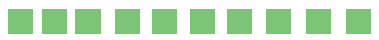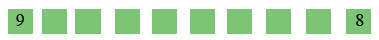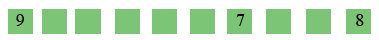SEARCH HOMEMath Central Quandaries & QueriesQuestion from Laxmi: 1)The integers  0 to 9 are  arranged along a straight line in some order .a) find a possible arrangement in which the sum  of any three adjacent numbers is less than 15.b)is there an arrangement of this kind 0 is not included in the numbers.Hi Laxmi,

I created 10 spots in a line, each to hold one of the 10 digits.The sum of any adjacent digits is to be small so I want the large digits to be spaced far apart. Hence I started with the 9 and the 8 in the two extreme locations.The next largest digit is 7 and I don't want it in any of the 3 positions adjacent to 9 or 8. I put it here.See if you can now place the 7 remaining digits.

For part b) if you have the nine digits 1 to 9 what is the sum of these digits?

Penny* Registered trade mark of Imperial Oil Limited. Used under license.Math Central is supported by the University of Regina and the Imperial Oil Foundation.